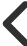Ideas Post### Collin Harness

@harnessmoney# How does interest work?

Understanding interest is important to creating or destroying wealth

## 1. Interest is the cost of borrowing money

As a consumer when you borrow money there is a cost to borrow those funds which is the interest rate.

The interest rate on credit cards can be anything from 1% to 30%.

Fixed or variable.

## 2. APY

The interest rate is always calculated on APY: Annual Percentage Yield. Yearly.

Whatever the rate is divide by 12. Interest is generally paid on a monthly basis.

## 3. Simple interest

A fixed amount each month based on the initial sum.

SI = P × R × T

P=Principal

R=Rate

T=Time

## 4. Compound interest

Every month as you receive an interest payment this amount is added to the principal to calculate the next month's interest amount. Over time your interest payments build.

A=P(1 + r/n)^nt

## 5. Savings accounts pay compound interest

Consumer lend money to banks and receive interest on their deposits. The interest paid to consumers is compound interest.

## 6. US Treasury bonds pay simple interest

When you buy a Treasury bond, bill or note it pays simple interest semi annually over a fixed period of time.

## 7. Series I bonds pay compound interest

There are special inflation bonds that pay compound interest.

## 8. The FEDs affect on interest rates

The fed funds rate impacts how much commercial banks charge each other for short-term loans. Banks use these funds to lend money to consumers. As the rate goes up it becomes more expensive for banks to borrow and banks become more conservative lending money to consumers.

0 Like.0 CommentBilland 2 more liked this01332500